# Standard paper sizes are an elegant example of simple maths

The well known A, B, C series paper sizes may seem arbitrary at first glance, but they are actually based on some simple basic principles which make it easy to calculate and understand. They are quite intuitive and easy to work with and are based on good mathematical foundations.

The single underlying premise for any standard paper size is extremely simple:

When a sheet is cut in half (by width), the aspect ratio should be maintained

Using just this statement we can figure out the required aspect ratio. Once we have that ratio, we can also figure out the actual sheet sizes for the different series.

To illustrate this principle, in the image below, we take a sheet of paper with height `x` and width `y`. It is cut width-wise, and one half is discarded. The remaining half is rotated. That new height and width should have the same ratio as the original piece of paper.## Calculate the ratio

Using the above image as reference, we can now calculate the ratio of an A0 paper.

Given a sheet with `x` height and `y` width, the next size down results in a ‘new’ sheet with `y` height and `x/2` width. And remember that the ratio must be maintained. Which means: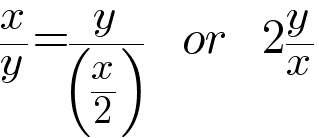Move the x and y across the equal sign, and we get: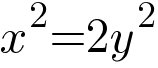Reducing it finally gives us the ratio,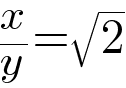Or in simplest terms, the ratio `x÷y = √2`.

The ratio of height to width of a standard sheet of paper is √2, or 1.414…

## Calculate the size of an A0 sheet

Within each series, the `0` size is the starting point, which is why we’ll start at size A0, as the B and C series definitions depend on it.

The A0 size has an additional property, which is:

The area of an A0 sheet is 1m2

That gives us the convenient formula `x*y=1`, and we can start substituting x as `1/y` and y as `1/x` in the above ratio.

We solve for x by substituting y=1/x.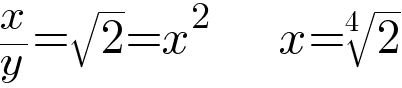Which is `1.1892071150...`

And solve for y by substituting x=1/y.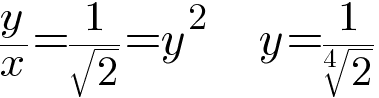Which is `0.8408964152...`

The answer - an A0 sheet is 0.841m wide and 1.189m tall.
As defined by the standard it’s 841mm x 1189mm.
If you multiply these however, you will get `999,949` which isn’t exactly 1m2 - this is due to the rounding necessary for instruments involved in the manufacturing and measuring process.

### Other A sheet sizes

At this point it should be pretty obvious: if we cut an A0 in half, we get an A1. If we halve an A1, we get an A2, and so on. The same applies to the B and C series.

We can now work our way down the remaining A sizes.

As illustrated earlier, the width of the previous size becomes the height of the next size. The height of the previous size is now halved.

Size Width Height
A0 841mm 1189mm
A1 `1189÷2=` 594mm 841mm
A2 `841÷2=` 420mm 594mm
A3 `594÷2=` 297mm 420mm
A4 `420÷2=` 210mm 297mm

This is an easy mental model to figure out paper sizes knowing the A0 starting point. For a proper equation for any given size, see Wikipedia

## B series sheets

The B series paper is used for posters, books and newspapers, and is meant for use when the A series is not ‘suitable’. Its sizes are related to the A series - each B size is the geometrical mean between adjacent sizes in the A series. The earlier principle of aspect ratio still remains, so we still have `x÷y = √2`. Furthermore, the width of a B0 sheet is set to 1000mm exactly.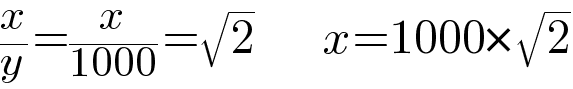B0 has a height of 1414mm and width of 1000mm.

As before, we can work our way down and figure out the remaining sizes.

Size Width Height
B0 1000mm 1414mm
B1 `1414÷2=` 707mm 1000mm
B2 `1000÷2=` 500mm 707mm
B3 `707÷2=` 353mm 500mm
B4 `500÷2=` 250mm 353mm

You can also verify these values as geometric means. For example, B1’s height will be the geometric mean between the heights of A0 and A1. That is, `√(841*594)`=707mm.

## C Series Sheets

C series sheets are meant for envelopes for A sheets, that is, a C4 envelope should be able to hold an A4 sheet without having to fold anything. A given C sheet size should be the geometric mean between its corresponding A and B sizes. As with others, the principle of aspect ratio still remains, so we still have `x÷y = √2`.

To figure out C0’s width, the geometric mean would be the square root of (A0’s width multiplied by B0’s width). `√(841*1000)` = 917mm. Similarly, C0’s height is `√(1189*1414)`=1297mm.

As before, we can work our way down and figure out the remaining sizes.

Size Width Height
C0 917mm 1297mm
C1 `1297÷2=` 648mm 917mm
C2 `917÷2=` 458mm 648mm
C3 `648÷2=` 324mm 458mm
C4 `458÷2=` 229mm 648mm

The three major paper series are done. In the event of civilizational collapse and loss of information we can reconstruct paper sizes, though the means and apetite for it may no longer exist.

## ISO Standard

The A, B, and C sizes are actually an international standard defined in ISO 216.

French professor Georg Lichtenberg was the first to propose the idea of using the √2 based aspect ratio. France was using A2 and A3 in the early 1800s and Germany further developed it in 1922 closer to the system we know today. It was then rapidly adopted by several countries and became a standard in 1975.

### Extensions

Various countries have additional variations or extensions on the international standard. The Swedish standards body SIS takes it further with their definitions of the D, E, F and G formats. Just like B and C, they are also geometric progressions between other sizes. Japan’s JIS has different roundings for sizes, and B series sheets are 1.5 times A series sheets, instead of √2. China adds a custom D series which is almost but not quite following the √2 ratio.

### Some paper sizes are arbitrary

As is customary with international standards, the US has its own separate specification for paper sizes, the US letter format, which Canada is also using as a de facto standard. The origins of the letter sizing is unknown and claimed to be a quarter of “the average maximum stretch of an experienced vatman’s arms”. The letter size was standardized to 8.5” x 11” in the 1980s.

Some countries such as Mexico, Chile, Columbia, and the Philippines, have officially adopted the ISO standard, but in practice use the US letter format.

Updated: